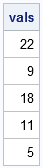A matrix is a convenient way to store an array of numbers. However, often you need to extract certain elements from a matrix. The SAS/IML language supports two ways to extract elements: by using subscripts or by using indices. Use subscripts when you are extracting a rectangular portion of a matrix, such as a row, a column, or a submatrix. Use indices when you want to extract values from a non-rectangular pattern.

### Extracting rows, columns, and submatrices

You can extract a submatrix by using subscripts to specify the rows and columns of a matrix. Use square brackets to specify subscripts. For example, if A is a SAS/IML matrix, the following are submatrices:

• The expression A[2,1] is a scalar that is formed from the second row and the first column of A.
• The expression A[2, ] specifies the second row of A. The column subscript is empty, which means “use all columns.”
• The expression A[ , {1 3}] specifies the first and third columns of A. The row subscript is empty, which means “use all rows.”
• The expression A[3:4, 1:2] specifies a 2 x 2 submatrix that contains the elements that are in the intersection of the third and fourth rows of A and the first and second columns of A.

The following SAS/IML statements demonstrate extracting submatrices:

```proc iml; A = shape(1:30, 5); /* 5 x 6 matrix */ scalar = A[2,1]; row = A[2, ]; /* 2nd row */ cols = A[ , {3 1}]; /* 3rd and 1st columns */ matrix = A[3:4, 1:2]; /* 2 x 2 matrix: (A[3,1] || A[3,2]) // (A[4,1] || A[4,2]) */ print A, scalar, row, cols, matrix;```

The previous examples were adapted from Wicklin (2013) "Getting Started with the SAS/IML Language", which I recommend for programmers who are starting to learn the SAS/IML language.

### Extracting diagonals and triangular elements

Non-rectangular patterns are common in statistical programming. Examples include the matrix diagonal and the lower triangular portion of a square matrix. The SAS/IML provides special functions for extracting diagonal and triangular regions:

For example, the following statements extract the main diagonal, the lower triangular elements in row-major order, and the lower triangular elements in column-major order:

```proc iml; S = shape(1:16, 4); /* 4 x 4 matrix */ v = vecdiag(S); L_row = symsqr(S); L_col = vech(S); print S, v, L_row, L_col;```

### Extracting arbitrary patterns of elements

For the extraction of arbitrary elements, you should use indices. SAS/IML software stores matrices in row-major order, which means the elements are enumerated as you move across the first row, then across the second row, and so forth. However, notice that you do not know the subscripts for A unless you know the shape of A. If A is a 3 x 3 matrix, A corresponds to A[1,3]. However, if A is a 2 x 2 matrix, A corresponds to A[2,1].

The SUB2NDX function enables you to convert subscript information into the equivalent indices. For example, suppose that B is a 5 x 5 matrix and you want to extract the following elements: B[5,2], B[2,4], B[4,3], B[3,1], and B[1,5]. The following statements convert the subscripts into indices and extract the elements:

```proc iml; B = shape(1:25, 5); /* 5 x 5 matrix */ subscripts = {5 2, 2 4, 4 3, 3 1, 1 5}; /* five (row,col) subscripts */ ndx = sub2ndx(dimension(B), subscripts); vals = B[ndx]; print vals;```A powerful advantage of indices is that you can use them to assign values as well as to extract values. For example, if v is a five-element column vector, the expression B[ndx]= v assigns the values v to the elements of B. Notice that this is a vectorized operation. If you do not use indices, you would probably write a DO loop that iterates over the subscripts. In general, vector operations are more efficient than looping operations.

The ability to access elements in an arbitrary order is a big advantage for SAS/IML programmers. Whereas the DATA step processes one observation at a time, the SAS/IML language enables you to access SAS data in whatever order makes sense for the algorithm that you are writing.

ShareDistinguished Researcher in Computational Statistics

Rick Wicklin, PhD, is a distinguished researcher in computational statistics at SAS and is a principal developer of SAS/IML software. His areas of expertise include computational statistics, simulation, statistical graphics, and modern methods in statistical data analysis. Rick is author of the books Statistical Programming with SAS/IML Software and Simulating Data with SAS.

1. Pingback: Flip it. Flip it good. - The DO Loop

2.how can I especifing a submatrix by removing a column and a row in a big matrix?
in R-project is posible put A[-i,-j] to do this

•3.Caleb Mwamburi on

How can one use the for loop and conditional execution commands to extract elements in the leading diagonal in a matrix?

•You can use the VECDIAG function to extract the main diagonal of a matrix. If that doesn't answer your question, please post your question (along with an example of what you have and want) to the SAS Support Community for IML.